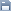Leetcode
2020.05.01 20:17

# 783. Minimum Distance Between BST Nodes

조회 수 768 추천 수 0 댓글 0
?

#### 단축키

Prev이전 문서

Next다음 문서

크게 작게 위로 아래로 댓글로 가기 인쇄
?

#### 단축키

Prev이전 문서

Next다음 문서

크게 작게 위로 아래로 댓글로 가기 인쇄

Given a Binary Search Tree (BST) with the root node `root`, return the minimum difference between the values of any two different nodes in the tree.

Example :

```Input: root = [4,2,6,1,3,null,null]
Output: 1
Explanation:
Note that root is a TreeNode object, not an array.

The given tree [4,2,6,1,3,null,null] is represented by the following diagram:

4
/   \
2      6
/ \
1   3

while the minimum difference in this tree is 1, it occurs between node 1 and node 2, also between node 3 and node 2.
```

Note:

1. The size of the BST will be between 2 and `100`.
2. The BST is always valid, each node's value is an integer, and each node's value is different.
3. This question is the same as 530: https://leetcode.com/problems/minimum-absolute-difference-in-bst/

```/**
* Definition for a binary tree node.
* public class TreeNode {
*     int val;
*     TreeNode left;
*     TreeNode right;
*     TreeNode(int x) { val = x; }
* }
*/
class Solution {
public int minDiffInBST(TreeNode root) {
List<Integer> list = new ArrayList<>();

queue.offer(root);
while(queue.size() > 0){
int size = queue.size();
for(int i=0; i<size; i++){
TreeNode node = queue.poll();
if(node.left != null){
queue.offer(node.left);
}
if(node.right != null){
queue.offer(node.right);
}

}

}

Collections.sort(list);

int minDiff = Integer.MAX_VALUE;
int prev = list.get(0);
for(int i=1; i<list.size(); i++){
int tmpDiff = Math.abs(prev - list.get(i));
if(tmpDiff < minDiff){
minDiff = tmpDiff;
}
prev = list.get(i);
}

return minDiff;
}
}```

```/**
* Definition for a binary tree node.
* public class TreeNode {
*     int val;
*     TreeNode left;
*     TreeNode right;
*     TreeNode(int x) { val = x; }
* }
*/
class Solution {
public int minDiffInBST(TreeNode root) {
List<Integer> list = new ArrayList<>();

traverseBST(root, list);

int minDiff = Integer.MAX_VALUE;
int prev = list.get(0);
for(int i=1; i<list.size(); i++){
int tmpDiff = Math.abs(prev - list.get(i));
if(tmpDiff < minDiff){
minDiff = tmpDiff;
}
prev = list.get(i);
}

return minDiff;
}

public void traverseBST(TreeNode node, List<Integer> list){
if(node == null){
return;
}

traverseBST(node.left, list);
traverseBST(node.right, list);
}
}```

?

 제목+내용제목내용댓글이름닉네임아이디태그
List of Articles
번호 분류 제목 글쓴이 날짜 조회 수
77 Leetcode 720. Longest Word in Dictionary 2020.05.07 928
76 Leetcode 844. Backspace String Compare 2020.05.05 824
75 Leetcode 697. Degree of an Array 2020.05.05 818
74 Leetcode 225. Implement Stack using Queues 2020.05.05 781
» Leetcode 783. Minimum Distance Between BST Nodes 2020.05.01 768
72 Leetcode 532. K-diff Pairs in an Array 2020.05.04 765
71 Leetcode 997. Find the Town Judge 2020.05.02 761
70 Leetcode 222. Count Complete Tree Nodes 2020.05.05 756
69 Leetcode 687. Longest Univalue Path 2020.05.01 756
68 Leetcode 235. Lowest Common Ancestor of a Binary Search Tree2020.05.01 756
67 Leetcode 724. Find Pivot Index 2020.05.05 751
66 Leetcode 605. Can Place Flowers 2020.05.05 736
65 Leetcode 56. Merge Intervals 2020.05.05 729
64 Leetcode 448. Find All Numbers Disappeared in an Array 2020.05.05 726
63 Leetcode 897. Increasing Order Search Tree 2020.05.04 725
62 Leetcode 872. Leaf-Similar Trees2020.05.04 719
61 Leetcode 876. Middle of the Linked List 2020.05.04 711
60 Leetcode 628. Maximum Product of Three Numbers 2020.05.05 705
59 Leetcode 203. Remove Linked List Elements 2020.05.04 697
58 Leetcode 270. Closest Binary Search Tree Value 2020.05.01 694
Board Pagination Prev 1 2 3 4 Next
/ 4